American Journal of Modern Physics
Volume 5, Issue 4-1, July 2016, Pages: 27-33

The Foundations of the Epistemology and the Methodology of Physics

Magi Mageshwaran1, Amrit Sorli2, Davide Fiscaletti3

1Physics department, St. Joseph's College, Bangalore, India

2Foundations of Physics Institute, Idrija, Slovenia

3SpaceLife Institute, San Lorenzo in Campo (PU), Italy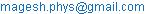(M. Mageshwaran)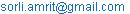(A.Sorli)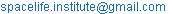(D. Fiscaletti)

Magi Mageshwaran, Amrit Sorli, Davide Fiscaletti. The Foundations of the Epistemology and the Methodology of Physics. American Journal of Modern Physics. Special Issue: Insufficiency of Big Bang Cosmology. Vol. 5, No. 4-1, 2016, pp. 27-33. doi: 10.11648/j.ajmp.s.2016050401.15

Received: April 27, 2016; Accepted: June 8, 2016; Published: June 30, 2016

Abstract: Three fundamental stones of physics are: observation of given phenomena, development of the mathematical model of observed phenomena, experiment which proves or disproves adequacy of the model with observed phenomena. In modern physics this fundamental methodology is sometime not taken in account. Certain models are confirmed as valuable picture of physical reality without that phenomena under examination would be observed. This represents serious problem which physics will need to take in account in order to preserve foundations of its methodology.

Keywords: Methodology of Physics, Epistemology, Gravity, Time, Cosmology, Higgs Field, Gravitational Waves

1. Introduction

The Merriam-Webster dictionary contains the following definition of epistemology: the study or a theory of the nature and grounds of knowledge especially with reference to its limits and validity. In this essay, we will examine how the foundations of physics are built. When searching for the foundations of physics it is important to look for an answer to this question: "Which fundamental elements of the universe are also fundamental elements of physics?". Let’s put ourselves in the position of an observer and see which are the fundamental elements of the universe. The first element is the observer himself. The second element is matter, the third element is energy, the fourth element is space in which matter and space exist. Matter and energy are permanently changing, so the fifth element is change. A given change has its appropriate duration, namely time as the sixth fundamental element of the universe: Observer, Matter, Energy, Space, Change and Time.

From Einstein we know that matter and energy are made out of the same "stuff", matter can transform into energy and, oppositely, energy can be transformed into matter. In physics, we call this intrinsic relationship between matter and energy the mass-energy equivalence principle and it is expressed by the famous equation: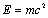. Matter and energy both exist in space.

During the 20th century, there was a conviction in physics that space is "empty" and without any physical property. In the General Theory of Relativity (GR), Einstein described gravity with a curvature of space. Einstein geometrised gravity. We will enquire now about the idea that space only has geometrical properties and is without physical properties from the point of view of epistemology. Let’s conduct an experiment: you observe a material object which is in front of you. Photons are reflected from that object, they come into your eye where they are transformed into an electrical signal which moves through nerves to the centre of the brain where the observer has an experience of the object. Your mind will elaborate information about the object which has reached the eye and so the experience will not be exactly the same as the perception. In epistemology, we call that an elaboration gap between perception and experience: perception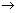elaboration (gap)experience. We need to answer the question: "Could experience of this material object happen in an empty space which has no physical attributes, can matter and energy exist in a space which only has a mathematical existence?". The idea of "empty space" as a fundamental arena is not falsifiable. The sentence: Matter and energy exist in an empty space is not falsifiable, which means it has no scientific value. It belongs to the philosophy of physics. In this book, we will establish a clear line between the philosophy of physics and the foundations of physics. We observe in physics that particles and antiparticles are continuously appearing from space and disappearing back into space. This experimental data shows that space has physical properties since, otherwise, physical particles could not appear from space and not disappear back into space. We can conclude that the physical elements of the universe, namely, matter and energy exist in space which is also physical; it has its physical properties, it is a kind of "energy".

2. Physical Properties of Space

Which are the physical properties of space energy? To answer this question, we will use Planck units which belong to the foundations of physics. Planck energy density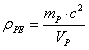is the average energy density of empty universal space in which there are no material objects. Out of this fundamental energy pool of the universe particles and antiparticles continuously appear and disappear. This picture in which matter and energy exist in the universal space which is a universal pool of energy is organic and rational. We can now rearrange our model of the universe in which we have four elements: Observer, Change, Time and Energy. To put this in a more mathematical form, we will call the universe set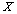which has three elements and one energy subset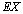: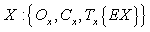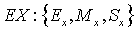,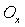-observer,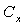-change,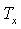-time,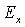-energy,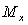-matter,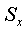-space .

In this picture, the appearance of particles and fields in the universe is no longer mysterious. The particles and fields have their origin in the energy of space. A given particle or massive body with mass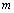and volume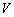is diminishing the energy density of space in its centre according to the following formula: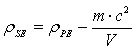.

From that formula, we can derive the formula for massof a given particle: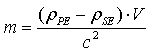.

The formula showing the whole relationship between energy, mass and the variable energy density of space regarding a given massive particle is as follows: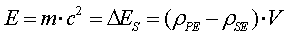The area of space with diminished energy density and the corresponding particle are a single phenomenon. We cannot divide the particle from the space in which the particle exists. The area of the diminished energy density of space gives the particle inertial mass and gravitational mass.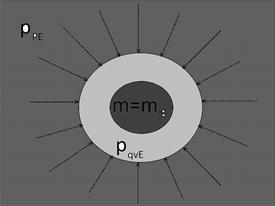Figure 1. Origin of mass.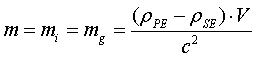When we have two or more particles together they create a common area of diminished energy density of space which generates gravity. Gravity force is the pressure of outer space with a higher energy density towards a lower space with less energy density.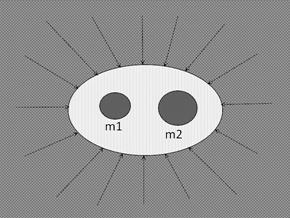Figure 2. Origin of gravity.

Two bodies are pushed together by the outer space pressure. There are no gravitons moving between the two bodies and carrying gravitational force. Gravitons are the biggest mistake of Einstein. Every big scientist makes big discoveries but also big mistakes. The result of my epistemological research is that mass and gravity are inherent physical proprieties of every massive particle and massive body and both have an origin in the diminished energy density of space caused by the presence of a given massive particle or massive body.

For 100 years we have been accustomed to thinking that space is empty. Now the idea that space is a fundamental pool of universal energy seems strange. How can we move in such a dense pool of energy? We know in physics that sound transmits in different materials much faster than in the air. The denser the material, the faster is the sound transmission. The same applies to space. The velocity of light in space depends on its density. The denser the space, the faster the light. The differences in speed are minimal, although we can measure them. Shapiro showed that light travels a little slower in space where gravity is stronger. This is because stronger gravity means a somewhat lower energy density of space.

3. Universe in Dynamic Equilibrium

The density of space in the entire universe is close to the Planck energy density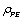. In the centre of black holes the energy density of space diminishes on a scale of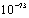, which means that a minimal variability of space is governing universal dynamics. In black holes and binary neutron stars, the density of space is below the minimum required for the stability of atoms and so atoms disintegrate into elementary particles and these disintegrate back into the energy of space. In outer intergalactic space, the energy density of space is at a maximum and the energy of space is continuously transforming into eliminatory particles. This circulation of energy is permanent, without a beginning or end.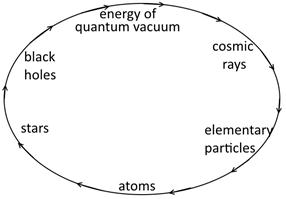Figure 3. Permanent circulation of energy in the universe.

In the above picture, energy of space is named energy of quantum vacuum, in this book we will stay with the term energy of space, which is more understandable. The universe is a non-created system in a permanent dynamic equilibrium and has no entropy. The increasing of the entropy we observe is only part of the universal cycle of energy. Black holes and neutron stars are recycling "old" matter in a fresh energy of space. This is the meaning of the ancient symbol of Ouroboros as we can see on figure 5.Figure 4. Snake eating its own tail.

We have seen that the energy of space is somehow fundamental. All different types of energy in the universe, including matter, are different forms of space energy. We can mathematically describe the universe as setwith three elements, a space energy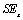subset which has two elements: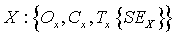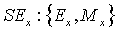.

The mass-energy equivalence principle allows us to see matter and energy as one physical entity. We can describe the universe as setwith four elements: Observer, Change, Time and Energy of space which has forms of energy and matter.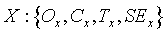Space energyis "missing" the dark energy of the universe. We see now that the universe is not so complicated. We have four fundamental elements of the universe: an Observer who is observing changes of energy. When measured with clocks, the changes are attributed with a duration. If there is no measurement from the side of the observer, there is no duration. Time exists only as a numerical order of changes. We distinguish between fundamental time which is a numerical order of changes and emergent time which is the duration of changes. The existence of duration requires measurement from the side of the observer.

4. Right Understanding of Time and Progress of Physics

The right understanding of time is the key for making progress in physics. In the model of this paper, element changes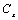, element time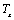and element space energyall have equal epistemological importance. Timeis the numerical order of changesof space energywhich the observer always experiences in the NOW. Strangely, in the universe there is always and only NOW. When you go into a lab and do experiments every day, you are doing them in the same NOW. There is nothing mysterious about NOW. We just have to acknowledge that the universe does not exist in some linear time made out of the past, present and future which are simply mind models through which the observer experiences the flow of changes occurring NOW. Our body is in NOW, information comes into the senses in NOW, then we elaborate them in the linear time model which is a basic mind frame in which we experience this timeless universe.

The space-time model of SR is not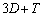. It is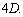The fourth coordinate of the Minkowski manifold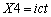is not temporal, it is spatial too.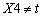. No signal can move in time, every signal moves in space only. The idea that CMBR has an origin in some remote temporal past is wrong. The CMBR signal has its origin in the universal energy pool of space energy.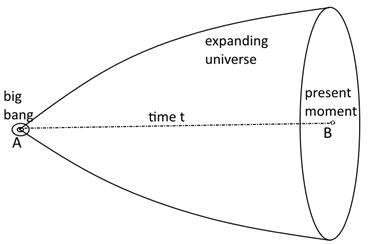Figure 5. Common imagine of Big Bang cosmology where time is presented as physical dimension.

This picture above which you can find in physics textbooks is wrong and has been deceiving physicists for the last few decades. The universe is NOW, always NOW and only NOW. Einstein has an intuition about this NOW: there is something essential about NOW which is just outside the realm of science. People like us, who believe in physics, know that the distinction between the past, present and future is only a stubbornly persistent illusion .

In the universe, element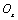is one which has not been studied much yet. Who is the observer is the fundamental question of physics. Among the four fundamental elements, the observer is the only one who is not changing. The observer is always the same and unchanged. You imagine that the observer would change. This would mean that every next day you would experience your experiment differently. Without stability of the observer, physics would not be possible. In every physicist, there is the same observer using the human body to experience this world. The origin of the observer is consciousness itself, which is omnipresent in the entire universe. The average scientific mind is against this idea. Big scientists of the calibre of Einstein and Planck were favourable to the idea that spirit-consciousness is the consistent part of the universe. Consciousness acts through the human body via the bio-photons and microtubules of the brain: consciousnessbio-photonsmicrotubulesnerve systemsenses .

Consciousness is not made out of "quanta", it is not matter or energy in a known sense of these terms. Today, physics is based on bivalent logic: a given phenomenon can be matter A or energy B. In mathematics, trivalent logic also exists where a given phenomenon can be in set A, set B or set C. Consciousness belongs to C. This view where the observer uses the human organism to experience the physical world puts the observer as a system at absolute rest. When we have two observers in two spaceships which pass close to each other moving at two different constant speeds, both observers adjust their clocks. Then after a few days they meet again. They look at the clocks. The spaceship in which the clock ticks slower has a higher speed than the spaceship in which the clock ticks faster. This "relative" velocity of clocks is valid for both observers. The body of the observer in the faster spaceship is ageing slower than the body of the observer in the slower spaceship.

GPS confirms that the "relative" velocity of clocks on satellites and on the ground is valid for all observers. In physics textbooks we have the famous example of an observer at a station and an observer on a train. For the observer at the station the clock on the train is running slower, while for the observer on the train the clock at the station is running faster. Each clock has its "local time" and is not valid for all observers. Besides that, we have in the Special Theory of Relativity (SR) also the "coordinate time" of each clock and, with the "dilation" of coordinate time, we are able to describe the constant velocity of light in all inertial systems. The Minkowski manifold is a mathematical model which manages to describe the constancy of light speed in all inertial systems, but it also leads to contradictions. In physics textbooks, we have a picture which shows how the "observer at rest" sees a moving vertical photon clock.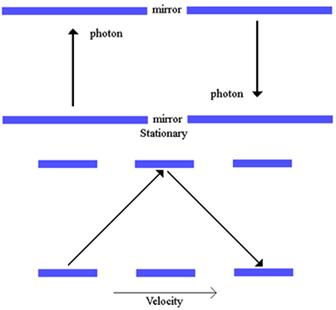Figure 6. Common imagine of rest and moving photon clock for stationary observer.

The official explanation is that the observer at rest sees the photon moving in a zig-zag motion and so the photon clock ticks slower for him because the photon needs more time. This is absolutely wrong because we know that this is only an optical illusion due to the motion which cannot prolong the photon path. The fact is that a stationary photon clock and a moving photon clock have the same rate for a stationary observer simply because light has a constant speed in all inertial systems. The reason light has a constant speed in all inertial systems is not only a postulate of SR, but has a deep physical origin, namely, light is a vibration of space in which all inertial systems move. This insight allows us to describe SR in a 3D Euclidean space using Galilean transformations for the spatial coordinates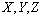and a Selleri transformation for time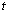. In addition, the second postulate of SR and Lorentz invariance has their roots in the minimal variability of the energy density of space in the entire universe.

5. Energy Density of Space and Equality of Inertial Mass and Gravitational Mass

Imagine you are in a spaceship in outer space far away from stellar objects. There is no gravity. When you start the engines and the spaceship is accelerating at a certain moment you have gravitational acceleration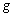exactly the same as on the Earth’s surface, namely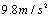. At this moment, the energy density of space in the spaceship is exactly the same as on the surface of the Earth. You calculate the energy density of space in the accelerating spaceship according to the following formula: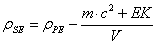,

whereis the mass of the spaceship,is its volume and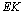is its kinetic energy. The accelerating spaceship is additionally absorbing the energy of space which accumulates in the spaceship as kinetic energy. If the spaceship were to crash into an asteroid, the kinetic energy would be released as heat and light.

We have seen that inertial mass and gravitational mass both have an origin in the diminished energy density of space. There is no need to search for the origins of the mass of particles because mass is an inherent property of particles with an origin in the diminished energy density of space. The Higgs mechanism is from this point of view a pure theoretical failure. The same is with the graviton. Gravity is an inherent property of particles and massive bodies having an origin in the area of the diminished energy density of space caused by the presence of a given particle or massive body. It would be hard for theorists to accept this model because it is not complicated enough. In theoretical physics of today, models about mass and gravity have become so complicated and complex that nobody really fully understands them. We have various schools which have their own different models. Physics has become a kind of philosophy. This situation has its roots in two fundamental misunderstandings of physics of the 20th century: 1. Universal space is empty and has no physical properties. 2. Time is a 4th dimension of space. Giving space its energy density and giving time its right meaning assures the progress of physics.

6. Curvature of Space, Density of Space and Dragging Effect

The curvature of space and the stress-energy tensor represent milestones of physics. The General Theory of Relativity (GR) is one of the jewels of physics. With my research fellow the Italian physicist Davide Fiscaletti we have shown that the curvature in space in SR has its origin in the variable energy density of a quantum vacuum. The denser the space, the less is its curvature. The less space is dense, the stronger is the curvature . Also the stress-energy tensor is deeply related to the energy density of space. The area of the diminished energy density in which stellar objects travel is moving with the objects and is also rotating with them. This is the so-called dragging effect. Planets of the solar system are moving on their orbits through space which is moved by the Sun. That’s why planets have precession. We calculate planets’ precession with our model and arrive at exactly the same results as Einstein, confirming that our model has a high correspondence with the physical reality .

Space is a direct medium of gravity and also a direct information medium of entangled particles. According to Newton gravity is immediate, in the GR gravity is immediate. The idea that gravity could be carried by some particle is a mistake of Einstein. Space is in direct contact between two objects and so can carry gravitational force between them. The gravity theorem says that gravity between objects A and B can be transported by element C only when element C has direct physical contact with A and B. The graviton does not satisfy the gravity theorem. All that exists in the universe is a form of space energy; everything is made out of space. In this sense, space is related with all elements which exist in it. Space has the ability to carry instantaneous information between entanglement particles. If this had been understood at the start of the 20th century, there would have been no EPR thought experiment. We now have a century long discussion about the existence of hidden variables which enables entanglement. By including space in physics as a concrete physical reality, gravity and entanglement are fully described and understood in detail.

Michelson and Morley’s preposition that the Earth is moving through a stationary ether was wrong. We replace here ether with space and we know that the area of the diminished energy density of space is moving and turning with the Earth . Light is a wave of space and that’s why the MM experiment produced a null result. In interstellar space, the MM experiment would probably not give a null result. The speed of light is close to constant in the entire universe, it has minimal variations which depend on the variable energy density of space which slightly changes its permittivity and permeability.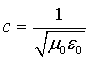In stronger gravity where space is less dense also the speed of light is minimally smaller. Shapiro measured this, but it was interpreted as a gravitational time dilation, which means that because of gravity the time coordinate of space is dilated and so light needs more time to move from point A to point B. Certainly, this interpretation is wrong because time is not the 4th dimension of space. Physicists are very sensitive to the idea that the speed of light cannot be absolutely constant, which is a kind of mental rigidity. Moreover, the idea that some particles such as neutrinos could have faster speed than light is seen as a kind of heretic attempt to demolish Einstein’s work. Even if it is proven that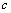is not the maximum speed in the universe, SR and GR will remain intact. The new model will also then include speed which is higher than.

In this universe, we have basic rules with some exceptions. The last few years have seen discussions about whether a neutrino is moving faster than light or not. However, it is not so important. In both cases, the Special Theory of Relativity (SR) and General Theory of Relativity (GR) remain stable grounds of physics. We have in the last few decades in physics a tendency to discover something new which will disprove old theories. Yet this will not happen because the grounds of physics are experiments, not theories which only describe them. Experiments are the primary tools of physics and theories are secondary tools of physics.

7. Time and Entanglement

A common understanding among the scientific community is that time stops at. This myth has no experimental basis and should be left behind. Every motion of space requires time as a numerical order of motion and when motion is measured as time as a duration. Motion happens in space only, not in time which is merely a mathematical parameter of motion in space where there is always NOW. Time is not a reality in which we live; time is a reality in which we think. As this is not yet clear, we now have in physics a new idea about time, namely, that time could have its origin in quantum entanglement. In the article (Moreva and others, 2014, Physical Review A) which presents this idea, an experiment is also proposed. It is nice that human fantasy has no limits, but in physics our ideas have to be controlled by epistemology which strictly excludes the possibility that time could have its origin in quantum entanglement. Further, the century-old discussions about travelling in time are categorically excluded. We can travel in space only and time is the duration of motion.

The myth that equations of physics should be time-symmetric should also be left behind. Imagination that time is like an infinite line where we are in the present moment at number zero which is now, the past is on the left side of the zero and future is on the right side of the zero has no correspondence in the physical world . In physics equations we have energy symmetry, where the amounts of energy on both the left and right sides of the equation are always equal. Also the idea that every particle should have a corresponding antiparticle does not seem exact. Feynman was thinking that when an electron is moving backward in time it becomes a positron. This is a classic example of how a brilliant physicist also makes mistakes. At his time, that idea that time was a 4th dimension of space was deeply rooted in physics.

In all equations of physics symbol of time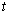represents fundamental time which is the numerical order of material changes, i.e. motion which run in space. When we measure numerical order with clocks we get duration which is emergent time. Considering time physical reality in which change run is epistemological misunderstanding which needs to be fully improved for further development of physics.

8. Syntropy is the primary Law of the Universe, Entropy is the Secondary Law of the Universe

With the development of the non-Euclidean geometries of Riemann and Lobachevsky the idea appeared that the universe might be finite. NASA results confirm that universal space has an Euclidean shape. This means that the universe is infinite and that the amount of space energy is infinite: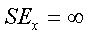.

We have two types of energy in the universe:

energy of spaceis primordial and syntropic energy which cannot be created and cannot be destroyed. Matter of the universe is structured energy of space and is ruled by entropy. The evolution of life on Earth is part of a universal process which runs in the entire universe and is developing towards the syntropic energy of the space. Matter has property of "self-organization" because it exists in space which is syntopic. Physical homogeneity of the universe also implies biological homogeneity. In the entire universe matter has a tendency to develop into intelligent conscious beings. Once we experience the origin of the observer, we become a self-conscious observer which is the same entity in every human being.

The energy of space is energy in its primordial form and is not made out of particles. Particles are made out of space energy, they are different structures of space energy. Photons are waves of space energy. Epistemological research confirms the universe has an ontological hierarchy as we can see in the figure below: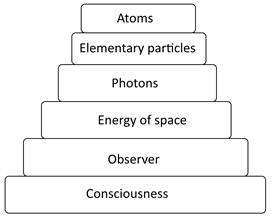Figure 7. Ontological structure of the universe.

The idea of an eternal universe ruled by syntropy is not familiar with the cosmological models prevailing today, although all experimental data are on the side of such an eternal universe. Man is born, he lives and dies. In physics, this human fact was projected in cosmology and so we have questions about the beginning of the universe which makes no sense. The universe is eternally NOW, linear time "past-present-future" belongs to the psychological time in which common observer experiences run of material changes which run in space where is always and only NOW. Observer has capacity to become aware of psychological time and to experience run of changes directly in NOW .

9. Fundamental Methodology of Physics

Physics methodological ground stones are next three:

1.   Observation of a given phenomena

2.   Mathematical model which describes the phenomena

3.   Experiment which proves or disproves adequacy of the model with observed phenomena.

Let’s take for example free fall: first physicists have observed free fall. After that they develop formalism which describes free fall. Finally they carried out experiments which confirm that formula F=mg is corresponding to the phenomena of freefall.

In "modern" physics this fundamental methodology is not always respected. For example confirmation of Higgs field with discovery of Higgs boson. Higgs boson discovery taken as experiment (3) confirms existence of mathematical model which describes Higgs field (2). But Higgs field was not observed. Ground stone (1) is missing. We develop a model where mass of a given particle is its intrinsic property. Our model does not require existence of Higgs field .

Other example is recent discovery of gravitational waves. LIGO has indirectly measured interference pattern of laser light (3). LIGO suspect that this interference pattern has physical origin in prolongation of one beam and shortening of other beam. LIGO suspect variability of beams length has physical origin in passing gravitational wave (2). Also here experimental data indirectly confirm mathematical model which predict that gravitational waves influence lengths of beams. Gravitational waves (1) were not observed. Our research group has a solution for LIGO results which is more plausible and excludes possibility that gravitational waves could influence lengths of beams .

10. Conclusions

Epistemology and methodology of physics are narrowing the gap between physical reality and correspondent mathematical models which describe it. In physics of 21 century the role of epistemology and methodology is under-valuated and might be that some theories which today seem stable and strong will be abolished in the next years because of their epistemological and methodological un-stability.

References

1. Fiscaletti, D. and Sorli, A.: "Bijective Epistemology and Space-Time", Foundations of Science, Springer, Volume 20,Issue 4, pp 387-398. (2015).
2. Amrit Sorli, Vlad Koroli, Andrei Nistreanu, Davide Fiscaletti.Cosmology of Einstein’s NOW. American Journal of Modern Physics. Special Issue: Insufficiency of Big Bang Cosmology. Vol. 5, No. 4-1, 2016, pp. 1-5. doi: 10.11648/j.ajmp.s.2016050401.1
3. Amrit Sorli. On the Origin of the Observer. American Journal of Modern Physics. Vol. 3, No. 4, 2014, pp. 173-177. doi: 10.11648/j.ajmp.20140304.14
4. Davide Fiscaletti, Amrit Sorli, Annales UMCS, Sectio AAA: PHYSICA.About a new suggested interpretation of special theory of relativity within a three-dimensional Euclid space, Volume 68, Issue 1, Pages 39–62, ISSN (Print) 0137-6861, doi: 10.2478/v10246-012-0018-1, March 2014
5. Fiscaletti D. and Sorli, A.: "Space-time curvature of general relativity and energy density of a three-dimensional quantum vacuum", Annales UMCS Sectio AAA: Physics 69, 55-81 (2015). doi: 10.1515/physica-2015-0004
6. Fiscaletti D. and Sorli A.: "Dynamic Quantum Vacuum and Relativity", Annales UMCS Sectio AAA, accepted for publication in August 2016
7. Magi Mageshwaran. Exact Solution for Time and Entanglement in UDE Cosmology. American Journal of Modern Physics. Special Issue:Insufficiency of Big Bang Cosmology. Vol. 5, No. 4-1, 2015, pp. 14-19. doi: 10.11648/j.ajmp.s.2016050401.13
8. A. Sorli, The Physics of NOW, Amazon (2014).
9. Amrit Sorli, Vlad Koroli, Andrei Nistreanu, Davide Fiscaletti, Magi Mageshwaran.UDE Cosmology Without Higgs Boson and Without Graviton. American Journal of Modern Physics. Special Issue: Insufficiency of Big Bang Cosmology. Vol. 5, No. 4-1, 2016, pp. 6-13. doi:10.11648/j.ajmp.s.2016050401.12
10. Amrit Sorli, Magi Mageshwaran, Davide Fiscaletti. Energy - Mass - Gravity Theory. American Journal of Modern Physics. Special Issue: Insufficiency of Big Bang Cosmology. Vol. 5, No. 4-1, 2016, pp. 20-26. doi: 10.11648/j.ajmp.s.2016050401.14

 Contents 1. 2. 3. 4. 5. 6. 7. 8. 9. 10.
Article ToolsAbstractPDF(2705K)